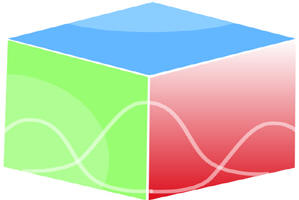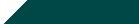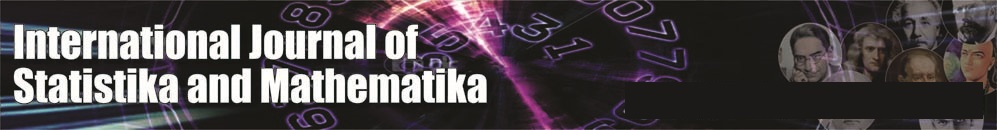``Home| Journals | Statistics Online Expert | About Us | Contact UsUntitled Document

On The Solution of Fractional Kinetic Equation

Chena Ram* and Palu Choudhary

Department of Mathematics and Statistics, Jai Narain Vyas University, Jodhpur-342005, Rajasthan, INDIA.

Research Article

Abstract: The object of this article is to study the fractional kinetic equation which  deals with certain problems in science and engineering. The results are obtained in a compact form containing Mittag-Leffler function, Robotov’s function and Hypergeometric function.

Keywords: Kinetic equation; Fractional operators; Mittag-Leffler function; Laplace transform; Hypergeometric function.

1.Introduction

The fractional kinetic equation  have importance in certain field of Physical phenomena governing diffusion in porous media, reaction and relaxtion processes in complex systems and anomalous diffusion etc. For detail , one can see  the monographs by Hilfer, Kilbas et al., Kiryakova and Podlubny . Fractional kinetic equations  are studied by Hille and Tamarkin, Glöckle and Nonnenmacher, Saichev and Zaslavsky, Saxena et al.[21-23]  and Zaslavsky.

The special function of the form

,   ,           (1.1)

was introduced by Mittag-Leffler, in 1903.

In 1905, this function was generalized by Wiman  as

,  Re(α)>0, Re(β)>0, .    (1.2)

Both functions are entire function of order ρ = and type σ = 1. The classical theory of these functions is presented in the handbook by Erdilyi et al.[6, section 18.1], while recent results are given in the Dzherbashyan[2,3]. Recently the interest to these functions has grown up by their application in some evolution problems.

Prabhakar introduced the function (z) as

,  Re(α), Re(β), Re(γ)>0, ,       (1.3)

where  is Pochhammer symbol [5, section 2.1.1] ,

= γ (γ+1) . . . . . . . . . . . .( γ+k-1)      ,    ( k = 1,2,3……)

and  = 1.

In 2007, Shukla and Prajapati  introduced the formula  (z) which is defined for  ; Re(α) , Re(β) , Re(γ) > 0 and q  (0,1)   N as :

,           (1.4)

where  =   denotes the generalized Pochhammer symbol.

The Mellin – Ross function  is defined as

.            (1.5)

Operators of fractional calculus have been studied by Srivastava and Saxena   . Due to their application in solution of integral and differintegral equation , the Riemann- Liouville operator of fractional calculus is defined by[14,16].

N(t) =   du ,         Re(α) >0 ,   (1.6)

with   N(t) = N(t) , and

=   N(t) ;   Re(α) , Re(n-α) > 0.         (1.7)

By using (1.6) and (1.7) , it yields that for N(t) =  , we have

=   ; Re(α) > 0, Re(>-1 , t >0,        (1.8)

and

=   , 0 < Re(α) <1 , Re (>-1 , t>0.        (1.9)

The standard kinetic equation ,

(t) = -   (t) ;                   >0.       (1.10)

By integrating standard kinetic equation , we obtain [9,p.58] ,

(t) -  (0) = -    (t) ,      (1.11)

where    is the standard Riemann integral operator. Here  (t) is the number density of species i, which is function of time (t) and  (t=0)= is the number density of species i at time t=0, .

The generalize form of (1.11)  by dropping index i , we obtain ,

N(t) -   = -   N (t) .                (1.12)

We can obtain more general integral equation than (1.12) by Laplace transform method by invoking the result given by Erdelyi et al. [4,p.182],

=  F(s),         (1.13)

where

= F(s) =  f(u) du , Re(s)>0.      (1.14)

Further, we use a fractional calculus method to obtain solution of differintegral equations related to fractional kinetic equation (cf.  ).

Our paper is devoted to further investigation of the fractional kinetic equation (1.11). The results are derived in a compact form by the application of Laplace transforms, which are suitable for numerical computation.

2. Laplace transform method:

The integral equation,

N(t) -  f(t)  = -   N (t),           (2.1)

it follows from (1.12) that ,

-  F(s) = -    ,

which reduces to

=     ,

provided s>c , we obtain the power series expansion as

=

now we apply inverse Laplace transform, then

N(t) =          (2.2)

where

* f(t) =   f(u) du ;      (2.3)

is convolution integral.

Main Results :

A number of  cases follows from (2.2) :

1. If  f(t) =   (µ ; _ ; -at ), then we can write convolution integral (2.3) as

*   (µ ; _ ; -at ) =      (µ ; _ ; -at ) du

=

=       du,

and substituting  x =  , which yields

=          dx,

=

The simplification of above equation gives ,

=         ,

(µ , µ ; mv+ µ ;-at ).     (2.4)

and by using (2.4) , integral equation (2.2) becomes ,

N(t) =        (µ , µ ; mv+ µ ;-at ),

N(t) =      (-)  (µ , µ ; mv+ µ ;-at ).              (2.5)

where ,   Re(a) , Re(mv+µ) > 0.

( II )  If  f(t) =    (v,a) =           (2.6)

where   (v,a) is Mellin Ross function defined by (1.5).

Then convolution integral equation (2.3) written as ,

* (v,a) =     (v,a) du ,

=

=     du ,

=       du ,

substituting x =  , we obtained

dx,

=      dx

on simplification of above equation ,

* (v,a) =               (2.7)

Now by using equation (2.7) in (2.2) , it becomes

N(t) =

If we assume that p = m , then

N(t) =

.              (2.8)

where  is  Robotov’s function .

We can write (2.8) in term of Mittag – Leffler function as ,

N(t) =     (-a,) .

N(t) =

.              (2.8)

where  is  Robotov’s function .

We can write (2.8) in term of Mittag – Leffler function as ,

N(t) =     (-a,) .

3. Special Case:

By (2.5) , we have

N(t) =      (-)  (µ , µ ; mv+ µ ;-at )

N(t) =      (-) ,

if we set k = 0 in above result , then we have

which is well  known result given by Saxena and Kalla.

References

1. B.N., Al-saqabi and V.K. Tuan, Solution of a Fractional Differential Equation, Integral Transf. Spec.Funct.4(4) (1966), 321-326.
2. M.M. Dzherbashyan, Integral Transforms and representations of Function in Complex     Domain (Russian) , Nauka , Moscow  (1966).
3. M.M. Dzherbashyan, Harmonic Analysis and Boundary Value Problem in the Complex Domain, Birkhaüser – Verlag ,Basel (1993).
4. A.Erdélyi, W. magnus, F. Oberhettinger and F.G.Tricomi, Tables of Integral Transform, Vol.II, Mcgraw-Hill, NewYork(1954).
5. A.Erdélyi, W. magnus, F. Oberhettinger and F.G.Tricomi, Higher Transcendental function, Vol.I, Mcgraw-Hill,NewYork-Toronto-London (1953).
6. A.Erdélyi, W. magnus, F. Oberhettinger and F.G.Tricomi, Higher Transcendental function, Vol.III, Mcgraw-Hill,NewYork-Toronto-London (1955).
7. W.G.Glöckle and T.F. Nonnenmacher , Fractional Integral Operators and Fox Function in the Theory of Viscoelasticity, Macromolecules 24(1991),6426-6434.
8. H.J.Houbold and A.M.Mathai,The Fractional Kinetic Equation and Thermonuclear Functions, Astrophys.Space Sci.273(2000),53-63.
9. R.Gorenflo and F.Mainardi, On Mittag-Leffler Function in Fractional Evolution, J.Comput . Appl.Math.,118(2000),283-299.
10. R.Hilfer(Ed.) , Applications of Fractional Calculus in Physics, World Scientific Publishing  Co.,NewYork (2000).
11. E.Hille and J.D. Tamarkin, On the Theory of Linear Integral Equations, Ann.Math.31(3), (1930),479-528.
12. A.A.Kilbas, H.M. Srivastava and J.J. Trujillo, Theory and Applications of Fractional Differential Equations, North-holland Mathematics Studies,204, Elsevier, Amsterdam (2006).
13. V.Kiryakova , Generalized Fractional calculus and Applications, John Wiley, NewYork (1994).
14. K.S.Miller and B.Ross, An Introduction to Fractional calculus and Fractional Differential Equations, John Wiley, NewYork (1993).
15. G.M.Mittag-Leffler , Sur la nouvelle function,C.R.Acad.Sci.Paris 137(1903),554-558.
16. K.B.Oldham and J.Spanier , The Fractional Calculus ,Acad. Press, NewYork (1974).
17. I.Podlubny, Fractional Differential Equations, Acad.Press , SanDiego (1999).
18. T.R.Prabhakar, A Singular Integral Equation with a Generalized Mittag-Leffler Function in Kernel, Yokohama.Math.J.19,(1971),7-15.
19. E.D. Rainville , Special Functions ,Macmillan – NewYork (1960).
20. A.Saichev and M. Zaslavsky , Fractional Kinetic Equations: solutions and Applications, chaos, 7(4)(1997),753-764.
21. R.K.Saxena ,A.M. Mathai and H.J.Haubold, On Fractional Kinetic Equations , Astrophys. Space Sci. 282(2002),281-287.
22. R.K.Saxena , A.M. Mathai and  H.J.Haubold, On Generalized Fractional Kinetic Equations ,Physica A 344(2004),653-664.
23. R.K.Saxena , A.M. Mathai and H.J.Haubold , Unified Fractional Kinetic Equation and a Fractional Diffusion Equation, Astrophys.Space Sci.290 (3/4) (2004),299-310.
24. R.K.Saxena and S.L.Kalla , On The Solution of Certain Fractional Kinetic Equations.Appl. Math. And Comput. 199(2008),504-511.
25. A.K. Shukla and J.C. Prajapati, On Generalized of Mittag-Leffler Function and its Properties, J.Math. Anal.Appl.336,(2007) 797-811.
26. H.M. Srivastava and R.K. Saxena , Operators of Fractional Integration and their Application, Appl. Math. Comput. 118(2001), 1-52.
27. A.Wiman, Über den Fundamental in der theorie der funktionen (x) , Acta.Math. 29(1905) 191-201.
28. G.M. Zaslavsky , Fractional Kinetic Equation for Hamiltonian Chaos, Physica D 78 (1-3) (1994)110-122.